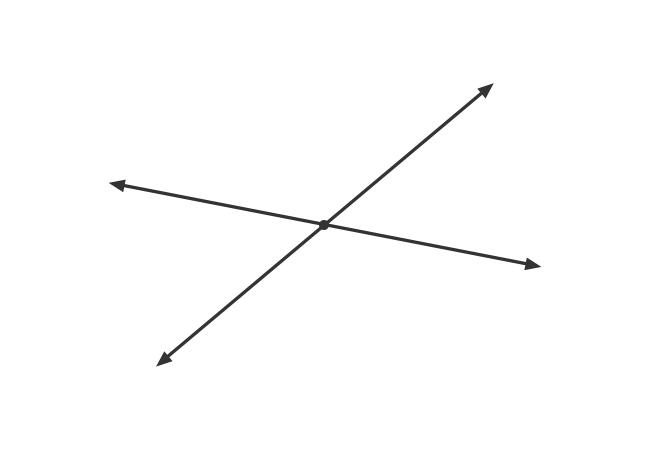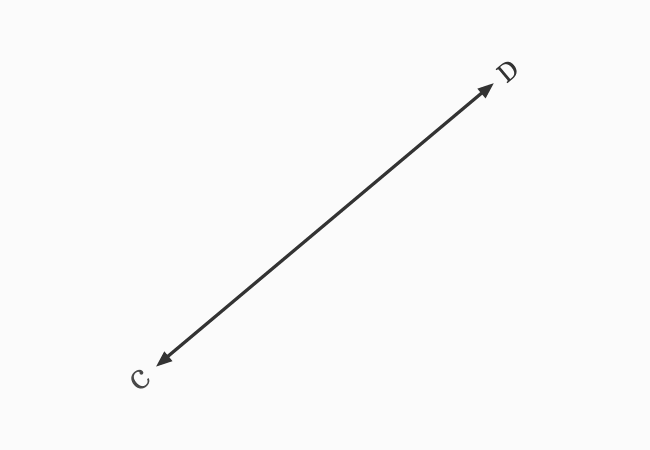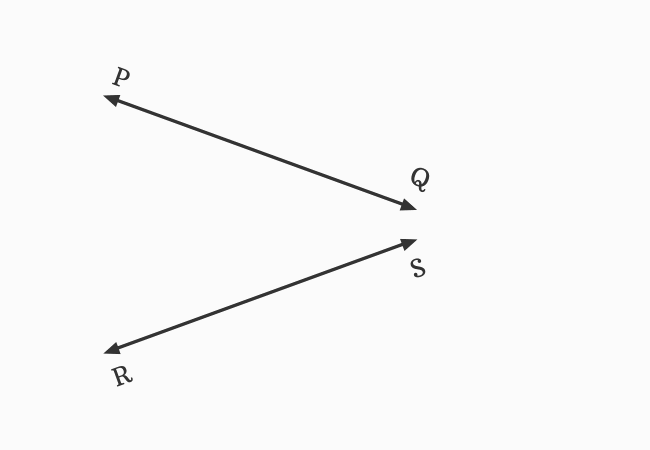# Intersecting lines

A straight line that divides another straight line at a point by passing is called an intersecting line.

## IntroductionIn English language, the word “intersect” means divide something by passing.

The travelling of one straight line definitely divides another straight line at a point for continuing its journey when two straight lines are non-parallel.

Due to their internal division at a point, one straight line is called an interesting line to the other straight line. Therefore, the two straight lines are called the intersecting lines.

### Examples

Look at the following two cases to learn the concept of intersecting lines with understandable examples.

#### Direct Intersection$\small \overleftrightarrow{AB}$ and $\small \overleftrightarrow{CD}$ are two straight lines, and they both meet each other at a point $I$ during their travelling. It seems, the straight line $\small \overleftrightarrow{AB}$ divides another straight line $\small \overleftrightarrow{CD}$ at point $I$, and vice-versa.

The straight line $\small \overleftrightarrow{AB}$ is called an intersecting line of $\small \overleftrightarrow{CD}$ and the straight line $\small \overleftrightarrow{CD}$ is called an intersecting line of $\small \overleftrightarrow{AB}$. Hence, the straight lines $\small \overleftrightarrow{AB}$ and $\small \overleftrightarrow{CD}$ are called the intersecting lines.

#### Extension for Intersection

The non-parallel lines cannot maintain equal distance between them at their corresponding opposite points. Therefore, the non-parallel lines always intersect in a plane at a point and they are called the intersecting lines.$\small \overleftrightarrow{PQ}$ and $\small \overleftrightarrow{RS}$ are two straight lines in a plane. It seems they do not meet each other at a point.

The picture shows that the straight lines $\small \overleftrightarrow{PQ}$ and $\small \overleftrightarrow{RS}$ are not parallel lines. So, they should meet each other at a point in a plane when they are extended until they meet each other.

Now, the straight lines $\small \overleftrightarrow{PQ}$ and $\small \overleftrightarrow{RS}$ are met at point $I$ to continue their journey. Hence, they are also called the intersecting lines.

Latest Math Topics
Jun 26, 2023
Jun 23, 2023

###### Math Questions

The math problems with solutions to learn how to solve a problem.

Learn solutions

Practice now

###### Math Videos

The math videos tutorials with visual graphics to learn every concept.

Watch now

###### Subscribe us

Get the latest math updates from the Math Doubts by subscribing us.